# Weighted colouring of hexagonal graphs.

 Importance: Medium ✭✭
 Author(s): McDiarmid, Colin Reed, Bruce A.
 Subject: Graph Theory » Coloring » » Vertex coloring
 Keywords:
 Posted by: fhavet on: March 13th, 2013
Conjecture   There is an absolute constantsuch that for every hexagonal graphand vertex weighting,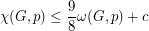A hexagonal graph is an induced subgraph of the triangular lattice. The triangular lattice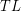may be described as follows. The vertices are all integer linear combinationsof the two vectorsand. Two vertices are adjacent when the Euclidean distance between them is 1.

Letbe a graph anda vertex weighting. The weighted clique number of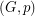, denoted by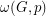, is the maximum weight of a clique, that is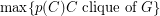, where. A-colouring of ais a mappingsuch that for every vertex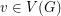,and for all edge,. The chromatic number of, denoted by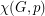, is the least integersuch thatadmits a-colouring.

The conjecture would be tight because ofthe cycle of length 9. The maximum size of stable set inis. Thusand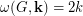, where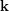is the allfunction.

McDiarmid and Reed [MR] proved that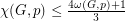for any hexagonal graphand vertex weighting. Havet [H] proved that if a hexagonal graphis triangle-free, then(See also [SV]).

The conjecture would be implied by the following one, whereis the allfunction.

Conjecturefor every hexagonal graph.

Since, whereis the stability number (the maximum size of a stable set). A first step to this later conjecture would be to prove the following conjecture of McDiarmid.

Conjecture   Letbe a triangle-free hexagonal graph.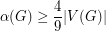## Bibliography

[H] F.Havet. Channel assignment and multicolouring of the induced subgraphs of the triangular lattice. Discrete Mathematics 233:219--231, 2001.

*[MR] C. McDiarmid and B. Reed. Channel assignment and weighted coloring, Networks, 36:114--117, 2000.

[SV] K. S. Sudeep and S. Vishwanathan. A technique for multicoloring triangle-free hexagonal graphs. Discrete Mathematics, 300(1-3), 256--259, 2005.

* indicates original appearance(s) of problem.